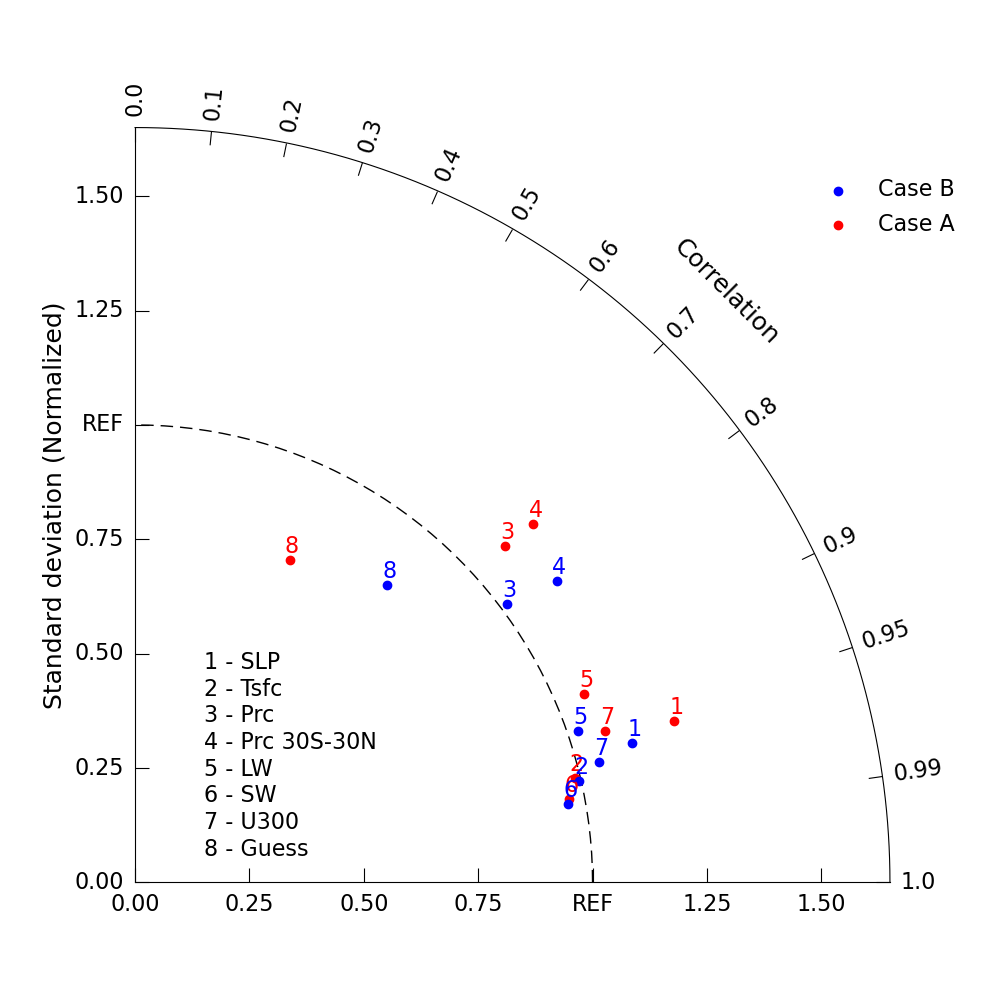# NCL_taylor_3.py#

This script illustrates the following concepts:
See following URLs to see the reproduced NCL plot & script:
Note: Due to limitations of matplotlib’s axisartist toolkit, we cannot include minor tick marks

between 0.9 and 0.99, as seen in the original NCL plot.

Import packages:

```import matplotlib.pyplot as plt

import geocat.viz as gv
```

Create dummy data:

```# Case A
CA_ratio = [1.230, 0.988, 1.092, 1.172, 1.064, 0.966, 1.079,
0.781]  # standard deviation
CA_cc = [0.958, 0.973, 0.740, 0.743, 0.922, 0.982, 0.952,
0.433]  # correlation coefficient

# Case B
CB_ratio = [1.129, 0.996, 1.016, 1.134, 1.023, 0.962, 1.048,
0.852]  # standard deviation
CB_cc = [0.963, 0.975, 0.801, 0.814, 0.946, 0.984, 0.968,
0.647]  # correlation coefficient
```

Plot:

```# Create figure and TaylorDiagram instance
fig = plt.figure(figsize=(10, 10))
dia = gv.TaylorDiagram(fig=fig, label='REF')

# Add models to Taylor diagram
CA_cc,
color='red',
marker='o',
label='Case A',
fontsize=16)

CB_cc,
color='blue',
marker='o',
label='Case B',
fontsize=16)

# Create model name list
namearr = ['SLP', 'Tsfc', 'Prc', 'Prc 30S-30N', 'LW', 'SW', 'U300', 'Guess']